﻿ Active Vibration Control in Engine Rotors using Electromagnetic Actuator System

Active Vibration Control in Engine Rotors using Electromagnetic Actuator System

M. Rajasekhar, Dr. J. SrinivasOPEN ACCESSPEER-REVIEWED

Active Vibration Control in Engine Rotors using Electromagnetic Actuator System

M. Rajasekhar1,, Dr. J. Srinivas2

1Department of Mechanical Engineering, Gitam Institute of Technology, Gitam University, Visakhapatnam, India

2Department of Mechanical Engineering, National Institute of Technology Rourkela, Rourkela, Orissa, India

Abstract

This paper presents active amplitude control methodology of a turbocharger rotor using the electromagnetic actuator design. Vibrations in such high-speed engine rotors are inevitable especially while crossing the critical operating speeds. From durability considerations, the rolling element and journal bearings are now-a-days replaced by compliant gas bearings. In present work, the rotor is discretized as a finite element model comprising three Timoshenko beam elements with consideration of gyroscopic effects. Unbalance and gravity are considered as the external forces. Dynamics response of the rotor is obtained by solving resultant dynamic equations using implicit time-integration scheme. An in-house program developed first computes critical operating states and with the help of existing theory of electromagnetic actuators, the vibration amplitudes of system are minimized by providing calculated additional external forces. Control led vibration amplitudes at a disk node is illustrated.

At a glance: Figures

1234
Prev Next

• Rajasekhar, M., and Dr. J. Srinivas. "Active Vibration Control in Engine Rotors using Electromagnetic Actuator System." Journal of Mechanical Design and Vibration 2.1 (2014): 25-30.
• Rajasekhar, M. , & Srinivas, D. J. (2014). Active Vibration Control in Engine Rotors using Electromagnetic Actuator System. Journal of Mechanical Design and Vibration, 2(1), 25-30.
• Rajasekhar, M., and Dr. J. Srinivas. "Active Vibration Control in Engine Rotors using Electromagnetic Actuator System." Journal of Mechanical Design and Vibration 2, no. 1 (2014): 25-30.

 Import into BibTeX Import into EndNote Import into RefMan Import into RefWorks

1. Introduction

Rotors under high speed operations such as turbochargers are often supported by the rigid surface bearings (ball bearings), gas foil bearings and floating ring bearings. Operating speed of a turbocharger rotor of a passenger car can go up to 200 krpm, which is much higher than any rotating industrial machines. Typically, such a rotor consists of bearing-supported rotor carrying compressor and turbine disks placed at two ends. Floating ring bearings are inexpensive and can provide a better damping effect with less frictional losses than conventional journal bearings. On the other hand, ball bearings are frictionless bearings that can be used in aero engine applications comfortably. Third category called gas foil bearings offer additional advantages over conventional ball bearings including high load capacity, low frictional heat generation and better stability at high speeds. They use working fluid (often air) as the lubricant, so that contamination due to lubricant leakage into working fluid can be eliminated. In principle, as the shaft rotates, a hydrodynamic pressure is generated between the shaft and top foil and the foil structures deform. This deformation will lead to a change in thickness of lubricant leading to bearing action. Characteristics of such a bearing performance can be determined by coupling the fluid film pressure with foil structural deformation. Many numerical and experimental studies have been carried out for understanding the behaviour of gas foils [1, 2, 3]. Recent works emphasized the importance of gas foils in rotor dynamics. Andres and Kim  modeled gas foil bearing force as third ordered structural element having nonlinear stiffness, which is derived from the measurements. Kim and Park  given the detailed description of design and construction of hydrodynamic air foil bearing system and tested at different speed and also compared the results with the published experimental data for the top foil deflection model. Zhou et al.  presented a dynamic analysis of a high speed turbo machinery rotor supported over viscoelastic support by altering the load and thickness of the bottom foil. Yu et al.  developed a numerical model of foil journal bearing by considering the deformation of foil structure and hydrodynamic pressure of lubricant film and is solved by FE method and then analyzed the characteristics of the bearing. Song and Kim  analyzed the new foil bearings, which is constructed with series of compression springs instead of bumps analytically and experimentally. Dykas and Howard  determined the imbalance corrections and temperature gradients of the journal due to foil bearing supports and also given design guidance to aid in failure prevention. More recently, Bhore and Darpe [10, 11] investigated nonlinear dynamics of a rotor supported over gas foil journal bearings using Reynolds equation for foil deformation. Application of gas foil bearings in high speed turbocharger rotors is not found anywhere in literature.

Vibration control of high speed rotors can be achieved effectively with active techniques. Recent literature provided several low cost solutions for amplitude attenuation of high speed rotors mounted on bearings including electromagnetic dampers  and electromagnetic exciters . More recently, Yu et al.  described the details of the control system for a flexible rotor bearing system using electromagnetic actuator. Sugai et al.  investigated the vibration control of a system supported over repulsive magnetic bearings while passing through the critical speeds.

Present work concentrates on the dynamic analysis of a rotor resting on foil bearing system and vibration control of the rotor using an electromagnetic (EM) actuator. The instability regions in the lateral vibration response of a flexible shaft system carrying rigid disc are initially predicted by considering both the rotating imbalance and nonlinear bearing forces. The control action in present task is provided by variation in electric current with respect to rotor displacements and velocities. The methodology is presented to show the effectiveness of EM actuator during crossing of critical speeds.

2. Mathematical Modeling

This section explains the shaft and bearing models considered in present work.

2.1. Finite Element Modeling of Rotor

A typical turbo compressor rotor is discretized into three elements as shown in Figure 1. The rotor has 4 nodes connected by 3 uniform circular beam sections. The disks are considered as rigid and rotor equations of motion are written as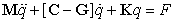(1)

where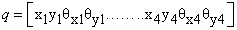is the displacement vector relating to the four nodes, M is distributed symmetric mass matrix, C is the system damping matrix known as viscous damping and G is the gyroscopic matrix of shaft, K denotes the stiffness matrix, F is external force vector consisting of gravity, unbalance and active force terms.

2.2. Rigid Disk

Each disk has four degrees of freedom, including two translations of mass center (x, y) and two rotations of the plane of disk (θx, θy). The finite element formulation of disk is written as: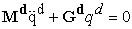(2)

The element mass and gyroscopic matrices corresponding to disk node are written as: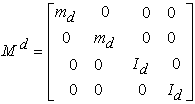(3)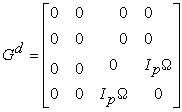(4)

Here, Id and Ip are respectively diametral (about x and y axes) and polar (about z-axis) moments of inertia of disk, while md is mass of disk and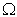is the speed of rotor.

2.3. Bearing

In linear bearing model, it is assumed that influence of slopes and bending moments as negligible. Both stiffness and damping at the bearings is considered in the model. Alternatively, the nonlinear bearing forces Fxb, Fyb due to foil bearing are calculated from the pressure field obtained on integration of time-dependent Reynolds equation. Foil bearing consists of three parts, (i) housing, (ii) bump foil that allows deformation of the bearing and (iii) top foil that generates a flat surface between the rotor and the bearing as shown in Figure 2. If the bearing lies at node i, the corresponding instantaneous bearing forces in x and y directions are given by: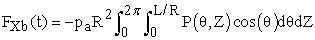(5)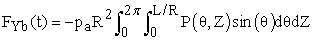(6)

Here, L is the bearing length, R is the bearing radius and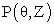is resultant normalized pressure field, pa is atmospheric pressure. The coordinates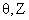denote the independent variables, which dictate gas film thickness, pressure and force components at every time instant. In bump foil radial bearing, the top foil is deflected by hydrodynamic pressure. It is assumed that the deflections of bumps in transverse direction are constant.

The pressure field is first obtained from integration of time- dependent Reynolds equation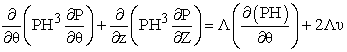(7)

where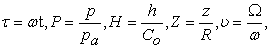(8)

where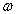is the angular velocity, pa is ambient pressure,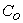is the radial clearance, h is the instantaneous film thickness (at attitude angle ) and is the function of initial geometry and foil deformation given by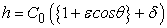(9)

Here, eccentricity ratio ε =e/C0 and dimensionless deflection of bump foil: δ = β× (P-1). Here, β is a compliance of bump foil written as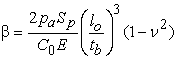(10)

The terms Sp,and tb are respectively bump pitch, half length and thickness of bump, while E and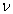are the elastic modulus and Poisson ratio of bump foil material.

For obtaining solution of Reynolds equation (7), often finite difference scheme is employed and the surface of bearing has been spread and nodal points have been set aside in the surface area (m +1) * (n +1). In the point with index (i, j), individual units of Reynolds equation are brought closer, replacing derivatives into following differential quotients (see Figure 3):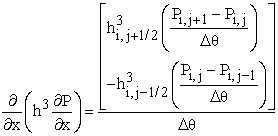(11)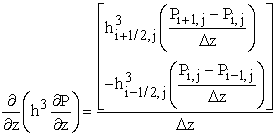(12)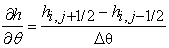(13)

After substituting into eq. (7) and organizing the terms, Pi,j can be obtained as: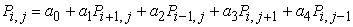(14)

where, a0, a1, a2, a3, a4 are constant data at each grid point. Pressure Pi, j is a function of these constants and is a function of four nearby pressure data in the grid. For n×m grid points, we get n×m equations, which can be solved by a computer program. For the points lying on the edges, the following boundary conditions are substituted: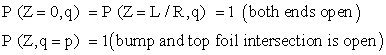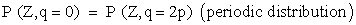(15)

3. Electromagnetic Actuator Model

The control system has an actuator placed very close to the plane of the disks and oriented along two orthogonal radial directions.

Due to the arrangement of poles in the actuator, the components of magnetic control force along X and Y directions are obtained independent of each other. The magnetic force is controlled by both the current and displacement. Tuning of current varies the stiffness of the rotor. Figure 4 shows schematic of four-pole electromagnetic actuator. The magnetic flux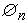for each pole is given by: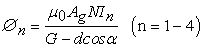(16)

where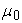denotes the permeability of free space (4π×10-7 H/m),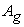be the effective cross sectional area of air gap, N the number of turns of winding coil around a pole, In the control current through the coil, G is the nominal gap size of air,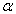is the half angle between the two adjacent poles (22.50) and d the displacement of shaft at the horizontal and the vertical directions. The magnetic force, Fn due to the flux density can be written as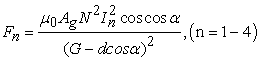(17)

From this equation, it is seen that the magnetic force is proportional to the square of the control current of an electromagnetic exciter and inverse to the displacement of a rotor. Fn is nonlinear function. For the sake of controlling, it is linearized. The PD controller first provides a bias current and then adds a small variable control current. Therefore, it is defined as: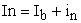(18)

Where Ib and in are the bias current and the control currents respectively. Replacing In (n= 1-4) in Eq. (15),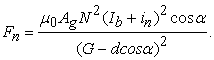(19)

Let i1= -i3 and i2= - i4 (see Figure 4) and then the magnetic forces in the x- and the y-direction are obtained as: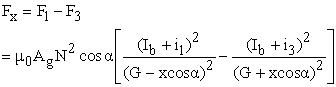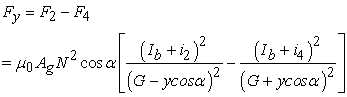(20)

The Taylor series is used to expand these equations. As the x- displacement and y-displacement and the control current in are small variances, the higher terms can be ignored in this equation. Consequently, the equation is obtained as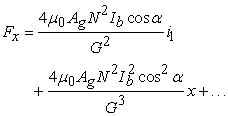(21a)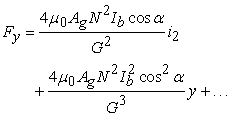(21b)

(Or)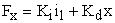(22a)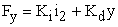(22b)

Where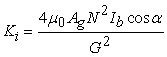, and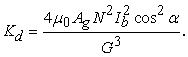Here, the currents i1 and i2 are given by: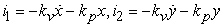(23)

where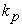and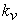as well as i1 and i2 are the proportional gains, the derivative gains and the currents of the controller in the x- and y-directions, respectively.

Figure 5 shows the block diagram of control system logic. The control strategy of the system described as follows. The input signals from the transducer, which is mounted on the ball bearing housing and the rotational speed signal (rpm) from the rotor are responsible for the magnetic force of an electromagnetic exciter. There might be remarkable change in the stiffness of the rotor system due to tuning of the current in the electromagnetic exciter. The Proportional - Derivative system controls the electromagnetic exciter and thereby changes the electrical energy to levitate the required position of the rotor system

Here the proportional and derivative gains kp and kv are selected as 10 and 0.1 respectively. Other parameters include: Ag=240 mm2, N=106, α=22.5o, G=0.9 mm and Ib =3 A. The instantaneous current components i1 and i2 depend on the displacements x and y at the disk node-1. Computer program is modified such that the right hand side force vector carries additional magnetic force components at the disk node.

4. Simulation Results

Linearly predicted unstable modes are helpful to obtain deeper insights into the nonlinear simulated results. For simplicity one can replace the gas foil bearing with constant stiffness isotropic spring damper in entire operating speed range. Table 1 shows the detailed data considered for the analysis of rotor.

Table 1. Geometric and Material data of the rotorDownload asPowerPoint Slide

Veiw figureView current table in a new window

Resulting assembled stiffness and mass matrices are obtained and the dynamic equations are solved for equivalent linear bearing counterpart with kxx=kyy=1e7 N/m for compressor side and kxx=kyy=1e8 N/m for turbine sides using Wilson-theta time integration scheme.

Figure 6. shows the multi-frequency unbalance response as the phase-diagram and Poincare map at compressor disk node, when the rotor is running at 500 rpm. It is seen that the motion is of period 3 non-synchronous vibratory nature.

As the rotor speed increases the motion becomes multi-periodic as seen in Figure 7. The motion becomes of quasi-period as the rotor speed reaches 40000 rpm as seen from Figure 8. To know more insights, it requires a bifurcation analysis with speed as parameter.

In order to minimize the vibration amplitudes at the compressor disk over the entire operating speed range, electromagnetic actuator is designed such that a null value of displacement and velocity should be achieved at the node. Here all the initial conditions are taken zero and effect of axial deformation is not considered during modeling.

Figure 9 shows the non-dimensional time response at the compressor node in the x- direction with and without control. It is clearly seen that initial chaotic motion has reduced in addition to amplitude reduction.

There is a huge difference in the amplitude of the motion, in the Figure 9 above half shows with electromagnetic exciter controlling and the lower half shows the time response at the disk position, when there is no controller. Amplitudes are falling down with aid of controller in the rotor bearing system.

Figure 10 shows the corresponding frequency response of the rotor computed with and without control. In absence of control, it is obvious that the rotor resonates at 450 Hz and after that the unstable motion persists due to the rotary force generated by internal damping of the shaft.

Figure 10. Amplitude reduction at critical speed without and with controller

5. Conclusions

In this study, a process of reduction in vibration amplitudes using electromagnetic actuators has been briefly illustrated using a small turbo-charger rotor system. The system has unbalance and gravity loading. Gyroscopic effects due to both shaft and disks were taken into account and unbalance was considered for both the disks. Initially, a flexible linear bearing system was considered for supporting the rotor and the implicit Wilson-theta time-integration scheme was used for solving the equations of motion. Phase diagrams, Poincare map and frequency response were used to access the instability in the system. As a future scope, the rotor has to be mounted on gas foil bearing at one end and ball bearing at other end to understand its effect on overall dynamics. An experimental system is also planned to show the effectiveness of control using electromagnetic excitation (actuator) system.

References

  Iordanoff,I., Said,B.B., Mezianne.A. and Berthier, Y, “Effect of internal friction in the dynamic behaviour of aerodynamic foil bearings”, Tribology International, 41 (5). 387-395.In article CrossRef  Crosby, W.A., “The incompressible lubrication of a ridged foil Bearing”, Wear, 113 (2). 247-266.In article CrossRef  Reddy, D.S.K., Swarnamani, S. and Prabhu, B.S, “Analysis of aerodynamic multi leaf foil journal bearings”, Wear, 209 (1-2). 115-122.In article CrossRef  Andres, L.S, and Kim, T.H, “Forced nonlinear response of gas foil bearing supported rotors” Tribology International, 41(8). 704–715.In article CrossRef  Kim, D. and Park, S, “Hydrostatic air foil bearings: Analytical and experimental investigation” Tribology International, 42(3). 413–425.In article CrossRef  Zhou, Q, Hou, Y. and Chen, C, “Dynamic stability experiments of compliant foil thrust bearing with viscoelastic support”, Tribology International, 42(5). 662-665.In article CrossRef  Yu, H., Shuangtao, C., Rugang, C., Qiaoyu, Z. and Hongli, Z. “Numerical study on foil journal bearings with protuberant foil structure” Tribology International, 44(9). 1061-1070.In article CrossRef  Song, J. and Kim, D., “Foil gas bearing with compression springs: Analyses and experiments” Transactions of the ASME, Tribology, 129 (3). 628-639.In article  Dykas, B. and Howard, S.A, “Journal Design Considerations for Turbo-machine Shafts Supported on Foil Air Bearings”, Tribology Transactions, 47 (4). 508-516.In article CrossRef  Bhore., S.P. and Darpe, A.K, “Investigations on characteristics of micro/meso scale gas foil journal bearings for 100–200 W class micro power systems using first order slip velocity boundary conditions and the effective viscosity model”, Microsyst Technol, 19 (4). 509-523.In article CrossRef  Bhore., S.P. and Darpe, A.K, “Nonlinear dynamics of flexible rotor supported on the gas foil journal bearings” Journal of Sound and Vibration, 332 (20). 5135-5150.In article CrossRef  Tonoli, A., Amati, N., Bonfitto, A., Silvagni, M., Staples, B. and Karpenko, E., “Design of Electromagnetic Dampers for Aero-Engine Applications” Journal of Engineering for Gas Turbines and Power, Trans.ASME, 132 (11). 112501-1-11.In article  Fan, C.C. and Pan, M.C, “Active elimination of oil and dry whips in a rotating machine with an electromagnetic actuator” International Journal of Mechanical Sciences, 53(2). 126-134.In article CrossRef  Yu, Z., Meng, L.T. and King, L.M. “Electromagnetic bearing actuator for active vibration control of a rotor” Proceedings of the IMechE, Part C: Journal of Mechanical Engineering Science, 212 (1). 705-716.In article  Sugai, T., Inoue T. and Ishida, Y. “Nonlinear theoretical analysis of contacting forward whirling vibration of a rotating shaft supported by a repulsive magnetic bearing” Journal of Sound and Vibration, 332 (11). 2735-2749.In article CrossRef  Fan, C.C. and Pan, M.C. “Fluid-induced instability elimination of rotor-bearing system with an electromagnetic exciter” International Journal of Mechanical Sciences, 52(4). 581-589.In article CrossRef  Tain, L., Wang, W.J. and Peng, Z.J. “Effect of bearing outer clearance on the dynamic behaviours of the full floating ring bearing supported turbocharger rotor” Mechanical Systems and Signal Processing, 31 (1). 155-175.In article